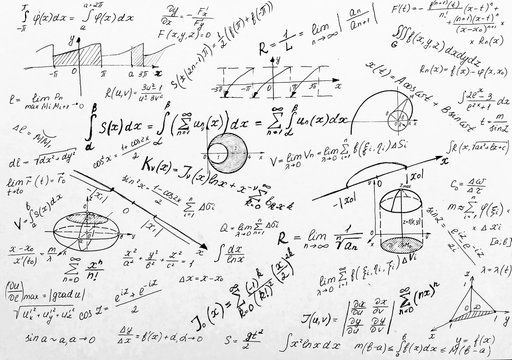# IB Math Analysis and Approaches

## Unlock the Power of Mathematics: Explore the Intriguing World of IB Math Analysis

"Mathematics is the universal language of logic and precision, guiding us in understanding the intricate patterns that shape our world." - UnknownWelcome to the captivating realm of IB Math Analysis, where you will embark on an exciting journey into the depths of mathematical concepts, reasoning, and problem-solving. This course offers a comprehensive exploration of the principles, techniques, and applications of mathematics.

Course Overview:

1. Algebra
2. Functions and Equations
3. Circular Functions and Trigonometry
4. Vectors
5. Statistics and Probability
6. Calculus
7. Exam Preparation and Review

Whether you have a passion for unraveling complex equations, exploring the intricacies of functions, or delving into the fascinating world of calculus, our IB Math Analysis program offers a dynamic and rigorous study of the subject at both Standard Level (SL) and Higher Level (HL).

Through a combination of theoretical study, problem-solving exercises, and mathematical exploration, students will develop a deep understanding of the fundamental concepts and applications of mathematics. IB Math Analysis nurtures logical reasoning, critical thinking, and the ability to apply mathematical principles in various contexts.

Course Topics:

1. Algebra: Explore the foundations of algebraic manipulation, solving equations, and the properties of functions.

2. Functions and Equations: Dive into the study of various types of functions, such as linear, quadratic, exponential, and logarithmic functions, and analyze their properties and transformations.

3. Circular Functions and Trigonometry: Unveil the intricate world of trigonometry, studying circular functions, identities, and their applications in solving real-world problems.

4. Vectors: Investigate the properties and applications of vectors, including vector operations, geometric interpretations, and their use in representing forces and motion.

5. Statistics and Probability: Analyze data, study probability concepts, and explore statistical methods for collecting, organizing, and analyzing data sets.

6. Calculus: Delve into the fascinating realm of calculus, exploring concepts such as limits, derivatives, integrals, and their applications in analyzing change, rates, and optimization problems.

In addition to the core topics, students will engage in problem-solving activities, mathematical investigations, and exploration of mathematical models. They will develop their analytical skills, logical reasoning, and critical thinking abilities through practical applications of mathematics.

In preparation for the IB Math Analysis exam, students will receive comprehensive support and guidance. They will practice exam-style questions, engage in problem-solving exercises, and review key concepts and mathematical techniques. By the end of the course, students will be well-prepared to demonstrate their understanding of mathematics and apply their knowledge to solve complex problems.

Through IB Math Analysis, you will unlock the power of mathematics, gain a deeper appreciation for its universal applications, and develop skills that are vital for analytical thinking and problem-solving.

Join us on this captivating journey into the fascinating world of IB Math Analysis, where you will unravel the patterns and structures that shape our world, explore the beauty of mathematical concepts, and nurture your potential as a confident and proficient mathematician.

"Mathematics is not about numbers, equations, or algorithms. It is about understanding." - William Paul Thurston